Get instant live expert help with Excel or Google Sheets“My Excelchat expert helped me in less than 20 minutes, saving me what would have been 5 hours of work!”

#### Post your problem and you'll get expert help in seconds

Your message must be at least 40 characters
Our professional experts are available now. Your privacy is guaranteed.

# Learn How to Generate Random Text Values in Excel

We can generate Random Text Values or Letters from a list by using the RANDBETWEEN and CHOOSE functions. This step by step tutorial will assist all levels of Excel users in generating random values between two numbers.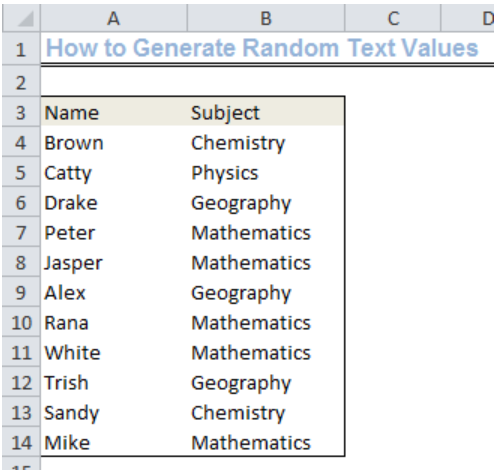Figure 1: Generated Random Text Values

## Setting up the Data

We will input the values as shown in figure 2 into Column A and Column B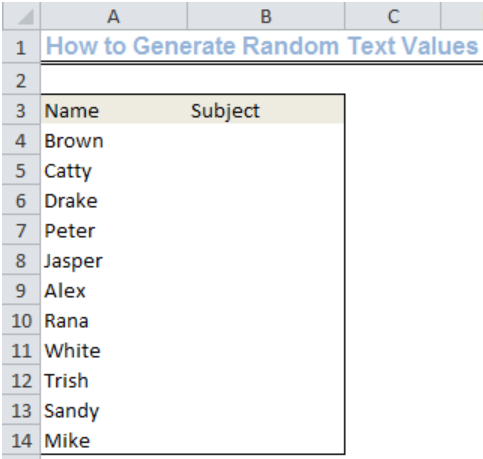Figure 2: Setting up the Data

## Syntax

`=CHOOSE(RANDBETWEEN(1,4),"Value1","Value2","Value3","Value4")`

## Explanation

• RANDBETWEEN function

We use the RANDBETWEEN function to generate an arbitrary number from a top and bottom value. This function uses the syntax,`=RANDBETWEEN(bottom, top)`, where the bottom value is the lowest value in the range and top is the highest value in the range.

RANDBETWEEN function returns only numbers and not text. This is why we need the CHOOSE function.

• CHOOSE function

We will use the CHOOSE function to return a value from our list of subjects in the formula. This function returns a value based on a specified index. Its syntax is, `=CHOOSE(index, val1, [val2], …[valn],)`.

The index is the position in the list where the value will be extracted from.

Val1, [val2], …[valn] is the list that is used.

## Formula

`=CHOOSE(RANDBETWEEN(1,4),"Chemistry","Physics","Geography","Mathematics")`

## Assigning Random Text Values

• We will click on Cell B4 and insert the formula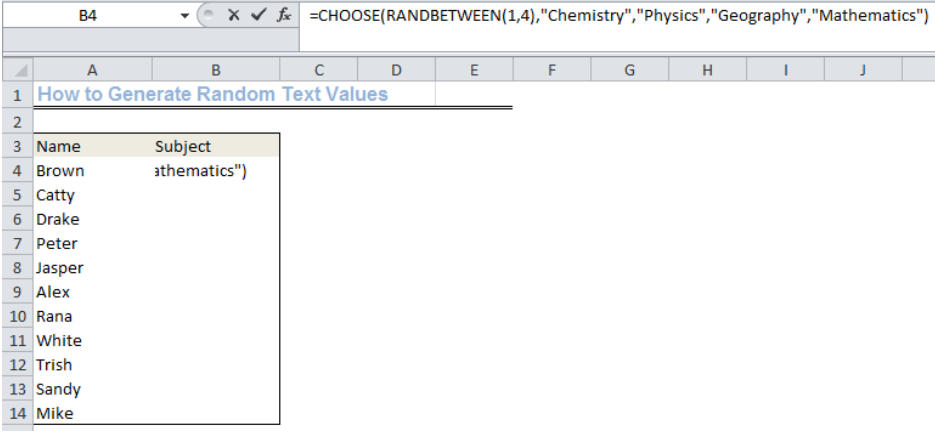Figure 3: Inserting the formula

• We will press enter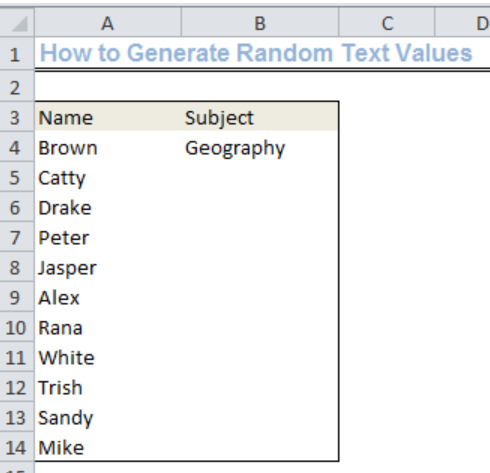Figure 4: First Random Text Value

• We will drag the formula from Cell B4 to B14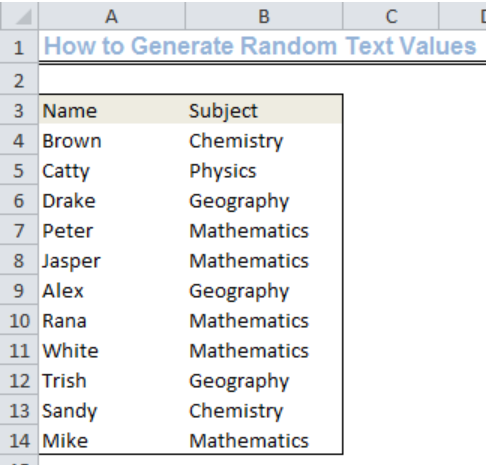Figure 5: Generated Random Text Values

## Note

The RANDBETWEEN function will generate a new value once we change our worksheet or exit it and return. This is the reason for the change in the value of Cell B4 in figure 4 and figure 5.

## Instant Connection to an Expert through our Excelchat Service

Most of the time, the problem you will need to solve will be more complex than a simple application of a formula or function. If you want to save hours of research and frustration, try our live Excelchat service! Our Excel Experts are available 24/7 to answer any Excel question you may have. We guarantee a connection within 30 seconds and a customized solution within 20 minutes.

### Did this post not answer your question? Get a solution from connecting with the expert.Another blog reader asked this question today on Excelchat:
Related blogs## Subscribe to Excelchat.coAnother blog reader asked this question today on Excelchat: